# Decimals Class 6 Extra Questions Maths Chapter 8

Decimals Class 6 Extra Questions Maths Chapter 8

### Decimals Class 6 Extra Questions Maths Chapter 8

Extra Questions for Class 6 Maths Chapter 8 Decimals

### Decimals Class 6 Extra Questions Very Short Answer Type

Question 1.
Write the following in decimals.
5 hundreds 3 tens 8 ones 4 tenths
Solution:Question 2.
Write 14.3 in place value table.
Solution:

 Hundreds Tens Ones Tenths 0 1 4 3

Question 3.
Write the following in decimals.Solution: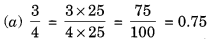Question 4.
Write the following as decimals.
(a) Three tens and eight-tenths
(b) Fifteen point seven
Solution:
(a) Three tens and eight-tenths = 3 x 10 + 8 x = 30 + 0.8 = 30.8
(b) Fifteen point seven = 15.7

Question 5.
Write the following as decimals.Solution:Question 6.
Write in decimals: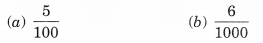Solution:Question 7.
Write as fractions in lowest form.
(a) 0.05
(b) 20.25
Solution:Question 8.
Write the following in decimals.
Three hundred six and seven hundredths.
Solution:
Three hundred six and seven hundredthsQuestion 9.
Which is greater 0.4 or 0.5?
Solution:Question 10.
Express the following as rupees using decimals:
(a) 7 paise
(b) 625 paise
Solution:### Decimals Class 6 Extra Questions Short Answer Type

Question 11.
Represent 1.3,3.8 and 4.1 on the number line.
Solution: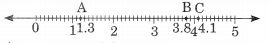A represents 1.3
B represents 3.8
and C represents 4.1

Question 12.
Read the numbers from the place value table and write them in decimals;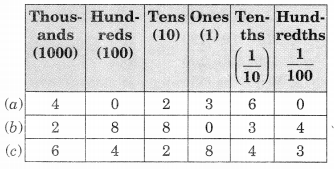Solution:Question 13.
Write each of the following as decimals:Solution:Question 14.
Convert the following unlike decimals into like decimals: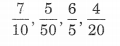Solution:
LCM of 10, 50, 5 and 20 = 100Question 15.
Write the following decimals in their expanded form:
(a) 27.65
(b) 102.05
(c) 36.36
(d) 0.507
Solution:
(a) 27.65(b) 102.05(c) 36.36(d) 0.507Question 16.
Write each of the decimals as a mixed fraction.
(a) 95.8
(b) 15.78
(c) 0.015
(d) 19.91
Solution:Question 17.
Express each of the following in terms of litres (L) using decimals:
(a) 625 mL
(b) 760 mL
(c) 11 L 125 mL
(d) 7 L 350 mL
Solution:Question 18.
Write 3.03, 2.75 and 2.5 in ascending order.
Solution:
The given decimals are unlike.
∴ Their corresponding like decimals are 3.03, 2.75 and 2.50.
Now neglecting the decimals, we have 303, 275 and 250.
Since, 303 > 275 > 250,
we have 3.03 > 2.75 > 2.50
∴ Ascending order is 2.50 < 2.75 < 3.03

Question 19.
Find the value of the following:
(а) 15 – 9.363
(B) 5.28 – 1.4 + 3.116
Solution:Hence, 5.28 – 1.4 + 3.116 = 6.996

### Decimals Class 6 Extra Questions Higher Order Thinking Skills (HOTS)

Question 20.
Mr. Ranjan purchased 15.500 kg rice, 25.750 kg flour and 3.250 kg sugar. Find the total weight of his purchases. Is it 50 kg or less? If less, how much less?
Solution:
Weight of rice = 15.500 kg
Weight of flour = 25.750 kg
Weight of sugar = 3.250 kg
Total weight of this purchases = 15.500 kg + 25.750 kg + 3.250 kg
= 44.500 kg
We see that the total weight of his purchases is less than 50 kg.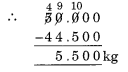Thus, the total weight 44.500 kg is 5.500 kg less than 50 kg.

Question 21.
Ten years old Rahul can carry a maximum weight of 15 kg. If he bought 4 kg 900 g of apples, 2 kg 600 g of grapes and 5 kg 300 g of mangoes. Can he carry the total weight that he bought. If yes, then how much more weight he can carry with him?
Solution:
Weight of apples = 4 kg 900 g
Weight of grapes = 2 kg 600 g
Weight of mangoes = 5 kg 300 g
∴ Total weight of his purchases = 4 kg 900 g + 2 kg 600 g + 5 kg 300 g
= 4.900 kg + 2.600 kg + 5.300 kg
= 12.800 kg
But Rahul can carry a maximum weight of 15 kg.
Thus more weight that he can carry with him = 15 kg – 12.800 kg= 2.200 kg

## SabDekho

The Complete Educational Website